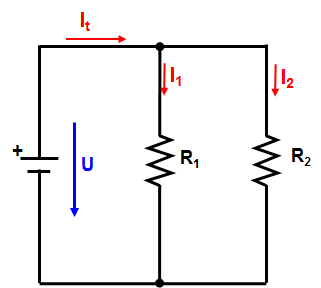Resistors parallel

### Resistors in Parallel - Parallel Connected Resistors

Laws of parallel connection

The voltage is the same everywhere in a parallel connection.

_________________________

The total current is the sum of all partial currents:

It =  I1  +  I2 + … +  In         (1)

________________________________

Determination of the total resistance:

It   =  I1  +  I2 + … +  I         Eq.(1) divided by V
V       V       V           V

according to the Law of Ohm follows

=      1    1   +    …   +     1
Rt           R1       R2                      Rn

Special case 1: All the resistors are of the same size

=         bzw.     Rt = R / n
Rt          R

Special case 2: Only 2 resistors are in parallel

=      1   +  1      =   R2 + R1
Rt             R    R2          R2 * R1

Form reciprocal and it follows:

Rt    R2 R1
R1  +   R2